Related Articles

# Operations on Sets

• Last Updated : 26 Nov, 2020

A set is a well-defined collection of objects. The objects may be numbers, alphabets, names of people, etc. Sets are represented using upper-case letters such as A, B, etc. For example:

A = {a, e, i, o, u}
A is a set of vowels in the English alphabet

### Three Important Points to Follow

The collection of objects must be well-defined. It means that we should be able to tell what belongs to the set and what does not. For example:

B = collection of good students is not a set
because we don’t know the criteria for good students. Thus there is some ambiguity as to
which students belong to the set and which do not.

Sets are written without duplicate elements. For example:

A = {1, 1, 2, 2, 3} must be written as A = {1, 2, 3}

The order of elements does not matter. For example:

A = {1, 2, 3} = {3, 2, 1}

## Representation

Sets can be represented in two ways

### Roaster form

In Roaster form, elements are listed between a pair of curly braces. The elements are separated by commas.

Example:

A = {a, e, i, o, u} is a set of vowels, represented in roaster form

### Set-builder form

In the set-builder form, a general element, and the common property the elements of the set are specified between a pair of curly braces.

Example:

A = {x: x is a vowel in the English alphabet}

here, x is the general element and the common property of elements is specified
after the colon (: symbol)

## Operations on Sets

### Intersection and Union of Sets

#### Intersection

The intersection of two sets A and B is a set that contains all the elements that are common to both A and B. Formally it is written asIn the following image, the shaded area is the intersection of sets A and BExample:

If A = {2, 3, 5, 7} and B = {1, 2, 3, 4, 5}
then the intersection of set A and B is the set A ∩ B = {2, 3, 5}

In this example 2, 3, and 5 are the only elements that belong to both sets A and B.

#### Union

Union of two sets A and B is a set that contains all the elements that are in A or in B or in both A and B. Formally it is written asIn the following image, the shaded area is the union of sets A and B.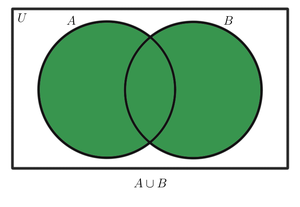Example:

If A = {2, 4, 8} and B = {2, 6, 8}
then the union of A and B is the set A ∪ B = {2, 4, 6, 8}

In this example, 2, 4, 6, and 8 are the elements that are found in set A or in set B or in both sets A and B

### Subset and Proper Subset

#### Subset

For two set A and B, A is a subset of B if every element in A is also in B. A can be equal to B. This is formally written asIn the following image, set A is a subset of B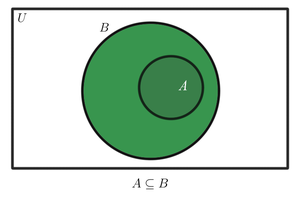Example:

If A = {2, 4} and B = {1, 2, 3, 4, 5, 6, 7, 8}
then A is a subset of B

In this example, A is a subset of B, because all the elements in A are also in B

Notes:

1. An empty set (or null set) is a subset of every set.Example:

∅ is a subset of the set {1, 2, 3, 4}

2. For a set A, the number of possible subsets is 2|A|. Where |A| = number of elements in A.

Example:

For the set C = {1, 2, 3}, there are 23 = 8 possible subsets
they are ∅, {1}, {2}, {3}, {1, 2}, {2, 3}, {1, 3}, {1, 2, 3}

#### Proper subset (also called strict subset)

For two sets A and B, A is a proper subset of B, if A is a subset of B and A is not equal to B. Formally it is written asExample:

For a set B = {1, 2, 3},
∅, {1}, {2}, {3}, {1, 2}, {2, 3}, {1, 3} are all proper subsets of B
Note that {1, 2, 3} is not a proper subset of B, because they are equal

### Superset and Proper Superset

#### Superset

For two sets A and B, if A is a subset of B then B is the superset of A. A can be equal to B. Formally it is denoted asIn the following image, set B is the superset of set A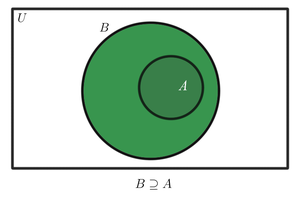Examples:

• If A = {2, 4} and B = {1, 2, 3, 4, 5, 6, 7, 8}
then B is the superset of A, because A is a subset of B

• If A = {11, 12} and B = {11, 12 } then B is the super set of A

#### Proper superset (also called strict superset)

For two sets A and B, if A is a subset of B and A is not equal to B, then B is the proper superset of A. Formally it is written asExamples:

• If A = {1, 2, 3} and B = {0, 1, 2, 3, 4, 5}
then B is a proper superset of A, because A is a subset of B and A ≠ B

• If A = {2, 4, 6} and B = {2, 4, 6} then B is not a proper superset of A, because A = B

### Relative Complement or Difference Between Sets

The relative complement or set difference of two sets A and B is the set containing all the elements that are in A but not in B. Formally this is written asSometimes this is also written as A \ B. In the following image, the shaded area represents the difference set of set A and set B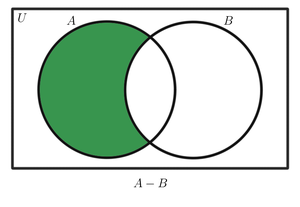Note:  A – B is equivalent to A ∩ B’ i.e.,  A – B = A ∩ B’

Example:

If A = {1, 2, 3, 4, 5, 6, 7, 8, 9, 10} and B = {2, 3, 5, 7}
then A – B = {1, 4, 6, 8, 9, 10}
and further B – A = ∅

### Universal Set and Absolute Complement

#### Universal set

A universal set is the set of all objects currently under consideration. It is usually denoted by the upper-case letter U. For example for a set of vowels, the universal set may be the set of alphabets.

Note: A set is always a subset of the universal set.#### Absolute complement

The absolute complement of a set A is the set of all elements that are in U but not in A. It is denoted as A’. In the following image, the shaded area represents the complement of set A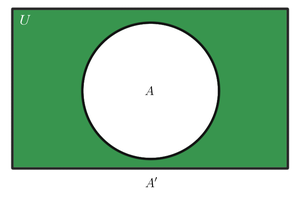The absolute complement is sometimes just called complement.

Note: A’ is equivalent to U – A i.e.,  A’ = U – A

Example:

If U = {1, 2, 3, 4, 5, 6, 7, 8, 9, 10} and A = {1, 2, 3}
then A’ = {4, 5, 6, 7, 8, 9, 10} = U – A

## Bringing the Set Operations Together

### De Morgan’s laws

1. The complement of the union of two sets is equal to the intersection of their complements
i.e.,  (A ∪ B)’ = A’ ∩ B’
2. The complement of the intersection of two sets is equal to the union of  their complements
i.e.,  (A ∩ B)’ = A’ ∪ B’

### Formula for the Cardinality of Union and Intersection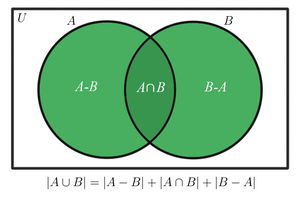The formula for the Cardinality of Union and Intersection is given below:

∣A ∪ B∣ = ∣A∣ + ∣B∣ − ∣A ∩ B∣

Proof:

We can write
|A ∪ B| = |A – B| + |A ∩ B| + |B – A|               —- by the sum of disjoint sets, refer to the Venn diagram above
|A ∪ B| = (|A| – |A ∩ B|) + |A ∩ B| + |B – A|    —- Substitute |A – B| = |A| – |A ∩ B|
|A ∪ B| = |A| + |B – A|                                    —- Simplify
|A ∪ B| = |A| + |B| – |A ∩ B|                            —- Substitute |B – A| = |B| – |A ∩ B|)

## Practical Problems on Union and Intersection of Two sets

Problem 1: There are 100 students in a class, 45 students said that they liked apples, and 30 of the students said that they liked both apples and oranges. Every student has to choose at least one of the two fruits. Find how many students like oranges.

Solution:

Let U = set of all students in the class
A = set of students that like apples
B = set of students that like oranges

Given:
|A| = 45
|A ∩ B| = 30
|U| = |A ∪ B| = 100 (because every student has to choose)

We need to find how many like oranges. i.e., |B|

The formula to be used is,
|A ∪ B| = |A| + |B| – |A ∩ B|        —-(i)

Subtract |A| – |A ∩ B| from both sides in (i) to get
|A ∪ B| – (|A| – |A ∩ B|) = |B|
or |B| = |A ∪ B| – (|A| – |A ∩ B|)

Substitute the given values and simplify,
|B| = |A ∪ B| – (|A| – |A ∩ B|)
= 100 – ( 45 -30 )
= 85

Thus the number of students that like oranges is 85.

Problem 2: There are a total of 120 students in a class. 70 of them study mathematics, 40 study science, and 10 students study both mathematics and science. Find the number of students who
i) Study mathematics but not science
ii) Study science but not mathematics
iii) Study mathematics or science

Solution:

Let,
U = set of all students in the class
M = set of students that study mathematics
S = set of students that study science

Our universal set here has 120 student i.e, |U| = 120

Given,
|M| = 70
|S| = 40
|M ∩ S| = 10  (number of students that study both mathematics and science)

i) Finding the number of students that study mathematics but not science. In the following image, the shaded area represents the set of students that study mathematics but not science.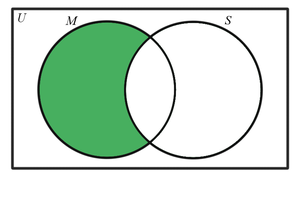We are required to find |M – S|
By the Venn diagram, we can see that |M – S| can be written as |M| – |M ∩ S|
thus,
|M – S| = |M| – |M ∩ S|
= 70 – 10
= 60

Thus the number of students who study mathematics but not science is 60

ii)  Finding the number of students that study science but not mathematics.  In the following image, the shaded area represents the set of students that study science but not mathematics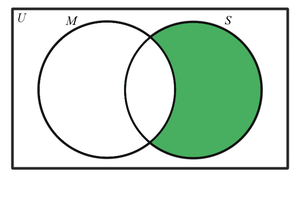We are required to find |S – M|
By the Venn diagram, we can see that |S – M| can be written as |S| – |M ∩ S|
thus,
|S – M| = |S| – |M ∩ S|
= 40 – 10
= 30

Thus the number of students who study science but not mathematics is 30

iii) Finding the number of students who study mathematics or science.  In the following image, the shaded area represents the set of students that study mathematics or science.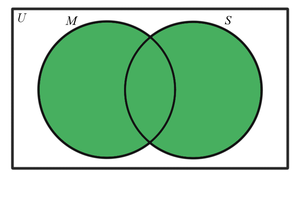We are required to find |M ∪ S|
By using the formula, |M ∪ S| = |M| + |S| – |M ∩ S|
|M ∪ S| = |M| + |S| – |M ∩ S|
= 70 + 40 – 10
= 100

Thus the number of students who study science or mathematics is 100.

Attention reader! Don’t stop learning now. Join the First-Step-to-DSA Course for Class 9 to 12 students , specifically designed to introduce data structures and algorithms to the class 9 to 12 students

My Personal Notes arrow_drop_up